Want to share your content on R-bloggers? click here if you have a blog, or here if you don't.

# Introduction

This article explains how to compute the main descriptive statistics in R and how to present them graphically. To learn more about the reasoning behind each descriptive statistics, how to compute them by hand and how to interpret them, read the article “Descriptive statistics by hand”.

To briefly recap what have been said in that article, descriptive statistics (in the broad sense of the term) is a branch of statistics aiming at summarizing, describing and presenting a series of values or a dataset. Descriptive statistics is often the first step and an important part in any statistical analysis. It allows to check the quality of the data and it helps to “understand” the data by having a clear overview of it. If well presented, descriptive statistics is already a good starting point for further analyses. There exists many measures to summarize a dataset. They are divided into two types: (i) location and (ii) dispersion measures. Location measures give an understanding about the central tendency of the data, whereas dispersion measures give an understanding about the spread of the data. In this article, we focus only on the implementation in R of the most common descriptive statistics and their visualizations (when deemed appropriate). See online or in the above mentioned article for more information about the purpose and usage of each measure.

# Data

We use the dataset iris throughout the article. This dataset is imported by default in R, you only need to load it by running iris:

dat <- iris # load the iris dataset and renamed it dat

Below a preview of this dataset and its structure:

head(dat) # first 6 observations
##   Sepal.Length Sepal.Width Petal.Length Petal.Width Species
## 1          5.1         3.5          1.4         0.2  setosa
## 2          4.9         3.0          1.4         0.2  setosa
## 3          4.7         3.2          1.3         0.2  setosa
## 4          4.6         3.1          1.5         0.2  setosa
## 5          5.0         3.6          1.4         0.2  setosa
## 6          5.4         3.9          1.7         0.4  setosa
str(dat) # structure of dataset
## 'data.frame':    150 obs. of  5 variables:
##  $Sepal.Length: num 5.1 4.9 4.7 4.6 5 5.4 4.6 5 4.4 4.9 ... ##$ Sepal.Width : num  3.5 3 3.2 3.1 3.6 3.9 3.4 3.4 2.9 3.1 ...
##  $Petal.Length: num 1.4 1.4 1.3 1.5 1.4 1.7 1.4 1.5 1.4 1.5 ... ##$ Petal.Width : num  0.2 0.2 0.2 0.2 0.2 0.4 0.3 0.2 0.2 0.1 ...
##  $Species : Factor w/ 3 levels "setosa","versicolor",..: 1 1 1 1 1 1 1 1 1 1 ... The dataset contains 150 observations and 5 variables, representing the length and width of the sepal and petal and the species of 150 flowers. Length and width of the sepal and petal are numeric variables and the species is a factor with 3 levels (indicated by num and Factor w/ 3 levels after the name of the variables). See the different variables types in R if you need a refresh. Regarding plots, we present the default graphs and the graphs from the well-known {ggplot2} package. Graphs from the {ggplot2} package usually have a better look but it requires more advanced coding skills. If you need to publish or share your graphs, I suggest using {ggplot2} if you can, otherwise the default graphics will do the job. All plots displayed in this article can be customized. For instance, it is possible to edit the title, x and y-axis labels, color, etc. However, customizing plots is beyond the scope of this article so all plots are presented without any customization. Interested readers will find numerous resources online. # Minimum and maximum Minimum and maximum can be found thanks to the min() and max() functions: min(dat$Sepal.Length)
##  4.3
max(dat$Sepal.Length) ##  7.9 Alternatively the range() function: rng <- range(dat$Sepal.Length)
rng
##  4.3 7.9

gives you the minimum and maximum directly. Note that the output of the range() function is actually an object containing the minimum and maximum (in that order). This means you can actually access the minimum with:

rng # rng = name of the object specified above
##  4.3

and the maximum with:

rng
##  7.9

This reminds us that, in R, there are often several ways to arrive at the same result. The method that uses the shortest piece of code is usually preferred as a shorter piece of code is less prone to coding errors and more readable.

# Range

The range can then be easily computed, as you have guessed, by substracting the minimum from the maximum:

max(dat$Sepal.Length) - min(dat$Sepal.Length)
##  3.6

To my knowledge, there is no default function to compute the range. However, if you are familiar with writing functions in R , you can create your own function to compute the range:

range2 <- function(x) {
range <- max(x) - min(x)
return(range)
}

range2(dat$Sepal.Length) ##  3.6 which is equivalent than $$max - min$$ presented above. # Mean The mean can be computed with the mean() function: mean(dat$Sepal.Length)
##  5.843333

Tips:

• if there is at least one missing value in your dataset, use mean(dat$Sepal.Length, na.rm = TRUE) to compute the mean with the NA excluded. This argument can be used for most functions presented in this article, not only the mean • for a truncated mean, use mean(dat$Sepal.Length, trim = 0.10) and change the trim argument to your needs

# Median

The median can be computed thanks to the median() function:

median(dat$Sepal.Length) ##  5.8 or with the quantile() function: quantile(dat$Sepal.Length, 0.5)
## 50%
## 5.8

since the quantile of order 0.5 ($$q_{0.5}$$) corresponds to the median.

# First and third quartile

As the median, the first and third quartiles can be computed thanks to the quantile() function and by setting the second argument to 0.25 or 0.75:

quantile(dat$Sepal.Length, 0.25) # first quartile ## 25% ## 5.1 quantile(dat$Sepal.Length, 0.75) # third quartile
## 75%
## 6.4

You may have seen that the results above are slightly different than the results you would have found if you compute the first and third quartiles by hand. It is normal, there are many methods to compute them (R actually has 7 methods to compute the quantiles!). However, the methods presented here and in the article “descriptive statistics by hand” are the easiest and most “standard” ones. Furthermore, results do not dramatically change between the two methods.

## Other quantiles

As you have guessed, any quantile can also be computed with the quantile() function. For instance, the $$4^{th}$$ decile or the $$98^{th}$$ percentile:

quantile(dat$Sepal.Length, 0.4) # 4th decile ## 40% ## 5.6 quantile(dat$Sepal.Length, 0.98) # 98th percentile
## 98%
## 7.7

# Interquartile range

The interquartile range (i.e., the difference between the first and third quartile) can be computed with the IQR() function:

IQR(dat$Sepal.Length) ##  1.3 or alternativaly with the quantile() function again: quantile(dat$Sepal.Length, 0.75) - quantile(dat$Sepal.Length, 0.25) ## 75% ## 1.3 As mentioned earlier, when possible it is usually recommended to use the shortest piece of code to arrive at the result. For this reason, the IQR() function is preferred to compute the interquartile range. # Standard deviation and variance The standard deviation and the variance is computed with the sd() and var() functions: sd(dat$Sepal.Length) # standard deviation
##  0.8280661
var(dat$Sepal.Length) # variance ##  0.6856935 Remember from this article that the standard deviation and the variance are different whether we compute it for a sample or a population (see the difference between the two here). In R, the standard deviation and the variance are computed as if the data represent a sample (so the denominator is $$n - 1$$, where $$n$$ is the number of observations). To my knowledge, there is no function by default in R that computes the standard deviation or variance for a population. Tip: to compute the standard deviation (or variance) of multiple variables at the same time, use lapply() with the appropriate statistics as second argument: lapply(dat[, 1:4], sd) ##$Sepal.Length
##  0.8280661
##
## $Sepal.Width ##  0.4358663 ## ##$Petal.Length
##  1.765298
##
## $Petal.Width ##  0.7622377 The command dat[, 1:4] selects the variables 1 to 4 as the fifth variable is a qualitative variable and the standard deviation cannot be computed on such type of variable. See a recap of the different data types in R if needed. # Summary You can compute the minimum, $$1^{st}$$ quartile, median, mean, $$3^{rd}$$ quartile and the maximum for all numeric variables of a dataset at once using summary(): summary(dat) ## Sepal.Length Sepal.Width Petal.Length Petal.Width ## Min. :4.300 Min. :2.000 Min. :1.000 Min. :0.100 ## 1st Qu.:5.100 1st Qu.:2.800 1st Qu.:1.600 1st Qu.:0.300 ## Median :5.800 Median :3.000 Median :4.350 Median :1.300 ## Mean :5.843 Mean :3.057 Mean :3.758 Mean :1.199 ## 3rd Qu.:6.400 3rd Qu.:3.300 3rd Qu.:5.100 3rd Qu.:1.800 ## Max. :7.900 Max. :4.400 Max. :6.900 Max. :2.500 ## Species ## setosa :50 ## versicolor:50 ## virginica :50 ## ## ##  Tip: if you need these descriptive statistics by group use the by() function: by(dat, dat$Species, summary)
## dat$Species: setosa ## Sepal.Length Sepal.Width Petal.Length Petal.Width ## Min. :4.300 Min. :2.300 Min. :1.000 Min. :0.100 ## 1st Qu.:4.800 1st Qu.:3.200 1st Qu.:1.400 1st Qu.:0.200 ## Median :5.000 Median :3.400 Median :1.500 Median :0.200 ## Mean :5.006 Mean :3.428 Mean :1.462 Mean :0.246 ## 3rd Qu.:5.200 3rd Qu.:3.675 3rd Qu.:1.575 3rd Qu.:0.300 ## Max. :5.800 Max. :4.400 Max. :1.900 Max. :0.600 ## Species ## setosa :50 ## versicolor: 0 ## virginica : 0 ## ## ## ## ------------------------------------------------------------ ## dat$Species: versicolor
##   Sepal.Length    Sepal.Width     Petal.Length   Petal.Width          Species
##  Min.   :4.900   Min.   :2.000   Min.   :3.00   Min.   :1.000   setosa    : 0
##  1st Qu.:5.600   1st Qu.:2.525   1st Qu.:4.00   1st Qu.:1.200   versicolor:50
##  Median :5.900   Median :2.800   Median :4.35   Median :1.300   virginica : 0
##  Mean   :5.936   Mean   :2.770   Mean   :4.26   Mean   :1.326
##  3rd Qu.:6.300   3rd Qu.:3.000   3rd Qu.:4.60   3rd Qu.:1.500
##  Max.   :7.000   Max.   :3.400   Max.   :5.10   Max.   :1.800
## ------------------------------------------------------------
## dat$Species: virginica ## Sepal.Length Sepal.Width Petal.Length Petal.Width ## Min. :4.900 Min. :2.200 Min. :4.500 Min. :1.400 ## 1st Qu.:6.225 1st Qu.:2.800 1st Qu.:5.100 1st Qu.:1.800 ## Median :6.500 Median :3.000 Median :5.550 Median :2.000 ## Mean :6.588 Mean :2.974 Mean :5.552 Mean :2.026 ## 3rd Qu.:6.900 3rd Qu.:3.175 3rd Qu.:5.875 3rd Qu.:2.300 ## Max. :7.900 Max. :3.800 Max. :6.900 Max. :2.500 ## Species ## setosa : 0 ## versicolor: 0 ## virginica :50 ## ## ##  where the arguments are the name of the dataset, the grouping variable and the summary function. Follow this order, or specify the name of the arguments if you do not follow this order. If you need more descriptive statistics, use stat.desc() from the package {pastecs}: library(pastecs) stat.desc(dat) ## Sepal.Length Sepal.Width Petal.Length Petal.Width Species ## nbr.val 150.00000000 150.00000000 150.0000000 150.00000000 NA ## nbr.null 0.00000000 0.00000000 0.0000000 0.00000000 NA ## nbr.na 0.00000000 0.00000000 0.0000000 0.00000000 NA ## min 4.30000000 2.00000000 1.0000000 0.10000000 NA ## max 7.90000000 4.40000000 6.9000000 2.50000000 NA ## range 3.60000000 2.40000000 5.9000000 2.40000000 NA ## sum 876.50000000 458.60000000 563.7000000 179.90000000 NA ## median 5.80000000 3.00000000 4.3500000 1.30000000 NA ## mean 5.84333333 3.05733333 3.7580000 1.19933333 NA ## SE.mean 0.06761132 0.03558833 0.1441360 0.06223645 NA ## CI.mean.0.95 0.13360085 0.07032302 0.2848146 0.12298004 NA ## var 0.68569351 0.18997942 3.1162779 0.58100626 NA ## std.dev 0.82806613 0.43586628 1.7652982 0.76223767 NA ## coef.var 0.14171126 0.14256420 0.4697441 0.63555114 NA You can have even more statistics (i.e., skewness, kurtosis and normality test) by adding the argument norm = TRUE in the previous function. Note that the variable Species is not numeric, so descriptive statistics cannot be computed for this variable and NA are displayed. # Coefficient of variation The coefficient of variation can be found with stat.desc() (see the line coef.var in the table above) or by computing manually (remember that the coefficient of variation is the standard deviation divided by the mean): sd(dat$Sepal.Length) / mean(dat$Sepal.Length) ##  0.1417113 # Mode To my knowledge there is no function to find the mode of a variable. However, we can easily find it thanks to the functions table() and sort(): tab <- table(dat$Sepal.Length) # number of occurences for each unique value
sort(tab, decreasing = TRUE) # sort highest to lowest
##
##   5 5.1 6.3 5.7 6.7 5.5 5.8 6.4 4.9 5.4 5.6   6 6.1 4.8 6.5 4.6 5.2 6.2 6.9 7.7
##  10   9   9   8   8   7   7   7   6   6   6   6   6   5   5   4   4   4   4   4
## 4.4 5.9 6.8 7.2 4.7 6.6 4.3 4.5 5.3   7 7.1 7.3 7.4 7.6 7.9
##   3   3   3   3   2   2   1   1   1   1   1   1   1   1   1

table() gives the number of occurences for each unique value, then sort() with the argument decreasing = TRUE displays the number of occurences from highest to lowest. The mode of the variable Sepal.Length is thus 5. This code to find the mode can also be applied to qualitative variables such as Species:

sort(table(dat$Species), decreasing = TRUE) ## ## setosa versicolor virginica ## 50 50 50 or: summary(dat$Species)
##     setosa versicolor  virginica
##         50         50         50

# Contingency table

table() introduced above can also be used on two qualitative variables to create a contingency table. The dataset iris has only one qualitative variable so we create a new qualitative variable just for this example. We create the variable size which corresponds to small if the length of the petal is smaller than the median of all flowers, big otherwise:

dat$size <- ifelse(dat$Sepal.Length < median(dat$Sepal.Length), "small", "big" ) Here is a recap of the occurences by size: table(dat$size)
##
##   big small
##    77    73

We now create a contingency table of the two variables Species and size with the table() function:

table(dat$Species, dat$size)
##
##              big small
##   setosa       1    49
##   versicolor  29    21
##   virginica   47     3

or with the xtabs() function:

xtabs(~ dat$Species + dat$size)
##             dat$size ## dat$Species  big small
##   setosa       1    49
##   versicolor  29    21
##   virginica   47     3

The contingency table gives the number of cases in each subgroup. For instance, there is only one big setosa flower, while there are 49 small setosa flowers in the dataset. Note that Species are in rows and size in column because we specified Species and then size in table(). Change the order if you want to switch the two variables.

Instead of having the frequencies (i.e.. the number of cases) you can also have the relative frequencies in each subgroup by adding the table() function inside the prop.table() function:

prop.table(table(dat$Species, dat$size))
##
##                      big       small
##   setosa     0.006666667 0.326666667
##   versicolor 0.193333333 0.140000000
##   virginica  0.313333333 0.020000000

Note that you can also compute the percentages by row or by column by adding a second argument to the prop.table() function: 1 for row, or 2 for column:

# percentages by row:
round(prop.table(table(dat$Species, dat$size), 1), 2) # round to 2 digits with round()
##
##               big small
##   setosa     0.02  0.98
##   versicolor 0.58  0.42
##   virginica  0.94  0.06
# percentages by column:
round(prop.table(table(dat$Species, dat$size), 2), 2) # round to 2 digits with round()
##
##               big small
##   setosa     0.01  0.67
##   versicolor 0.38  0.29
##   virginica  0.61  0.04

# Barplot

Barplots can only be done on qualitative variables (see the difference with a quantative variable here). A barplot is a tool to visualize the distribution of a qualitative variable. We draw a barplot on the qualitative variable size:

barplot(table(dat$size)) # table() is mandatory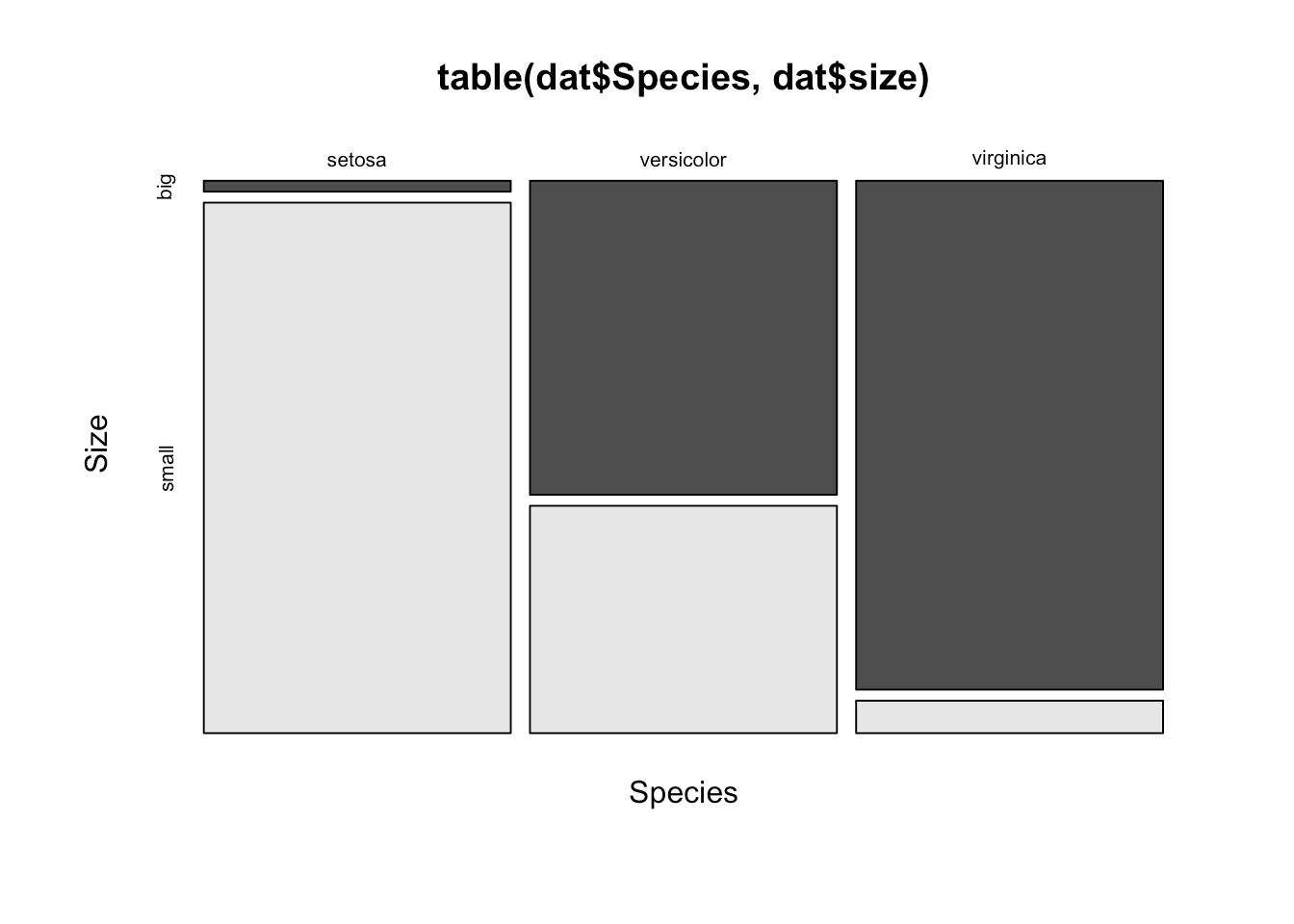You can also draw a barplot of the relative frequencies instead of the frequencies by adding prop.table() as we did earlier: barplot(prop.table(table(dat$size)))In {ggplot2}:

library(ggplot2) # needed each time you open RStudio
# The package ggplot2 must be installed first

ggplot(dat) +
aes(x = size) +
geom_bar()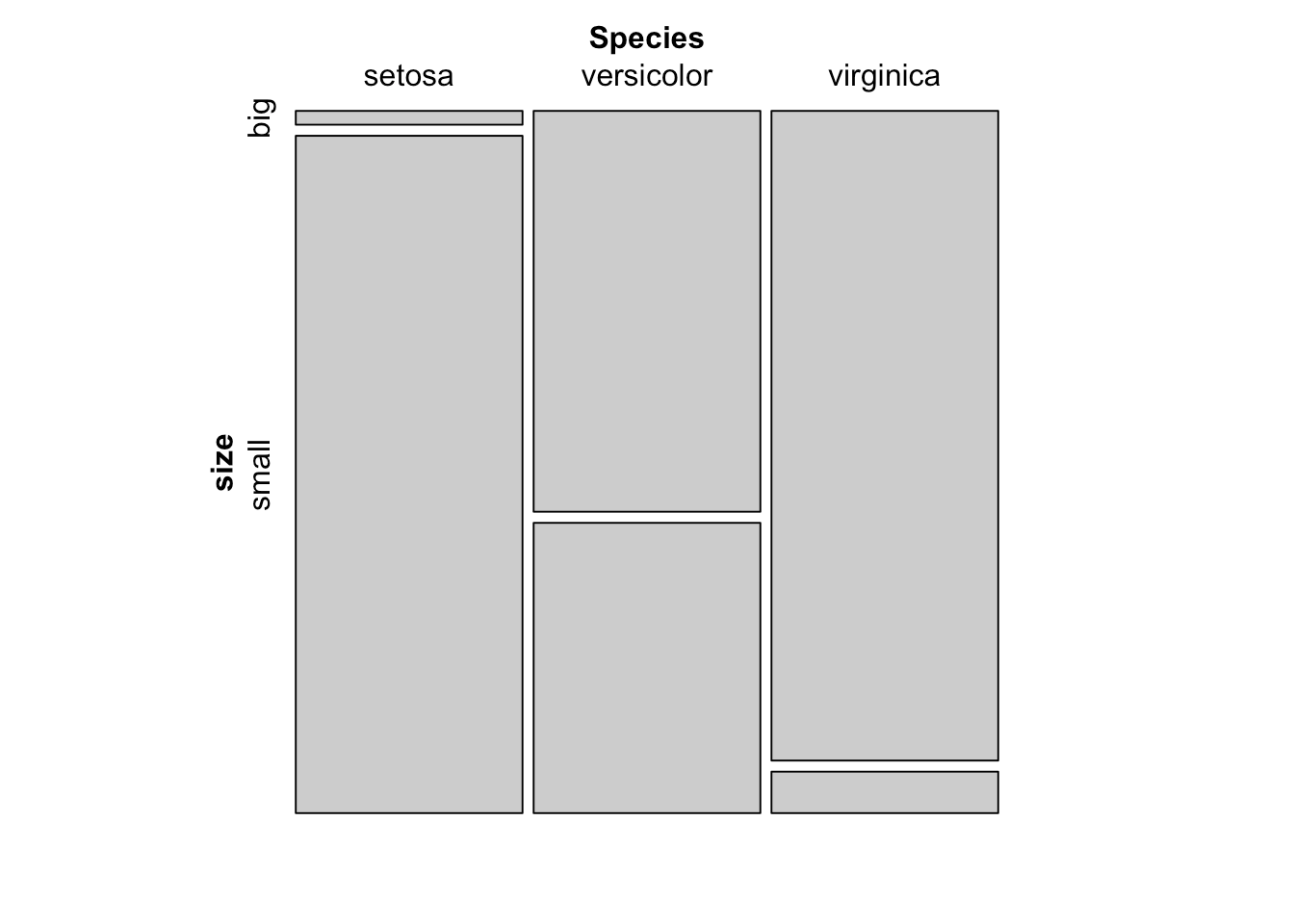# Histogram

A histogram gives an idea about the distribution of a quantitative variable. The idea is to break the range of values into intervals and count how many observations fall into each interval. Histograms are a bit similar to barplots, but histograms are used for quantitative variables whereas barplots are used for qualitative variables. To draw a histogram in R, use hist():

hist(dat$Sepal.Length)Add the arguments breaks = inside the hist() function if you want to change the number of bins. A rule of thumb (known as Sturges’ law) is that the number of bins should be the rounded value of the square root of the number of observations. The dataset includes 150 observations so in this case the number of bins can be set to 12. In {ggplot2}: ggplot(dat) + aes(x = Sepal.Length) + geom_histogram()By default, the number of bins is 30. You can change this value with geom_histogram(bins = 12) for instance. # Boxplot Boxplots are really useful in descriptive statistics and are often underused (mostly because it is not well understood by the public). A boxplot graphically represents the distribution of a quantitative variable by visually displaying five common location summary (minimum, median, first and third quartiles and maximum) and any observation that was classified as a suspected outlier using the interquartile range (IQR) criterion. The IQR criterion means that all observations above $$q_{0.75} + 1.5 \cdot IQR$$ and below $$q_{0.25} - 1.5 \cdot IQR$$ (where $$q_{0.25}$$ and $$q_{0.75}$$ correspond to first and third quartile respectively) are considered as potential outliers by R. The minimum and maximum in the boxplot are represented without these suspected outliers. Seeing all these information on the same plot help to have a good first overview of the dispersion and the location of the data. Before drawing a boxplot of our data, see below a graph explaining the information present on a boxplot:Detailed boxplot. Source: LFSAB1105 at UCLouvain Now an example with our dataset: boxplot(dat$Sepal.Length)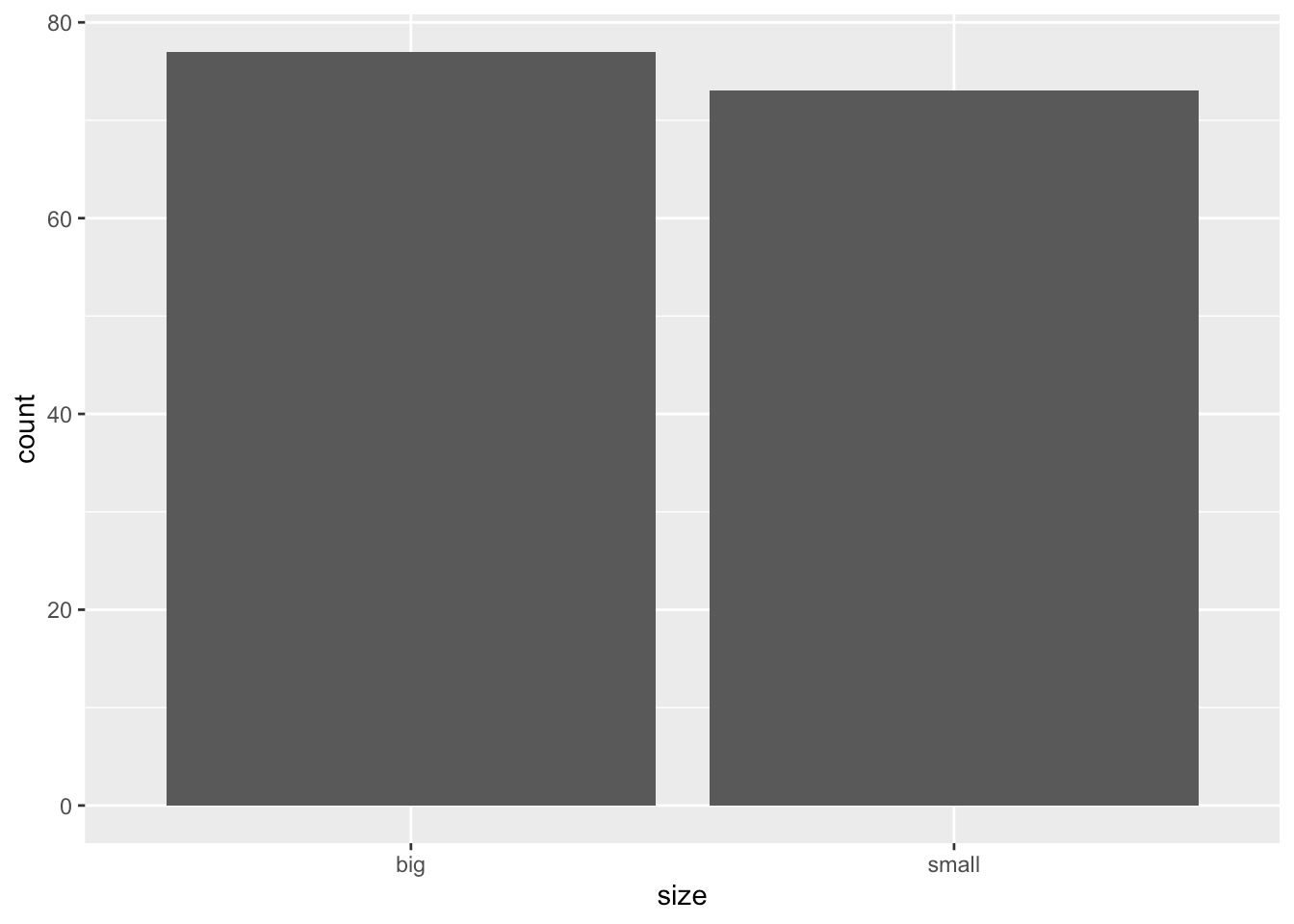Boxplots are even more informative when presented side-by-side for comparing and contrasting distributions from two or more groups. For instance, we compare the length of the sepal across the different species:

boxplot(dat$Sepal.Length ~ dat$Species)In {ggplot2}:

ggplot(dat) +
aes(x = Species, y = Sepal.Length) +
geom_boxplot()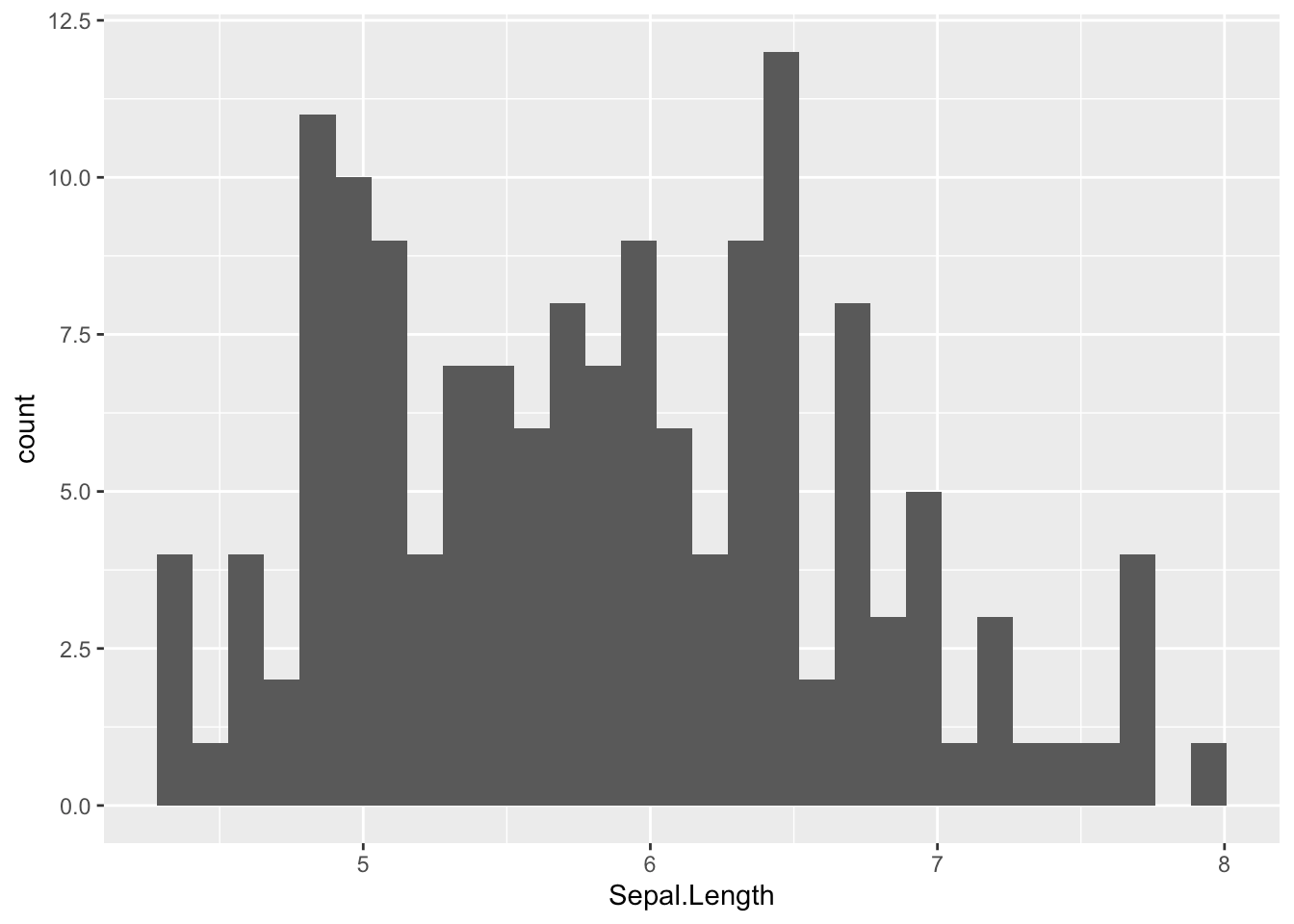# Scatterplot

Scatterplots allow to check whether there is a potential link between two quantitative variables. For instance, when drawing a scatterplot of the length of the sepal and the length of the petal:

plot(dat$Sepal.Length, dat$Petal.Length)There seems to be a positive association between the two variables.

In {ggplot2}:

ggplot(dat) +
aes(x = Sepal.Length, y = Petal.Length) +
geom_point()As boxplots, scatterplots are even more informative when differentiating the points according to a factor, in this case the species:

ggplot(dat) +
aes(x = Sepal.Length, y = Petal.Length, colour = Species) +
geom_point() +
scale_color_hue()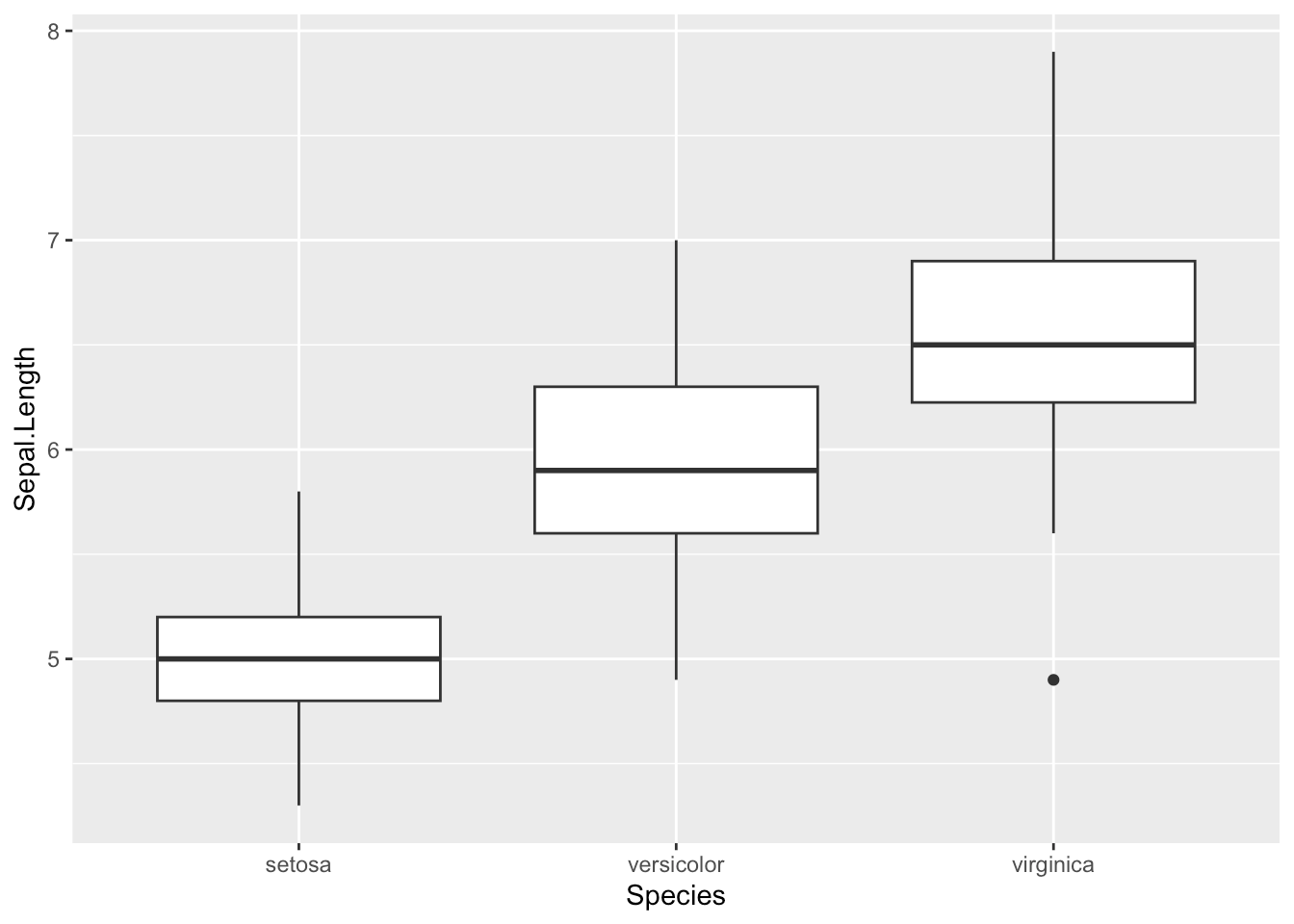# QQ-plot

## For a single variable

In order to check the normality assumption of a variable (normality means that the data follow a normal distribution, also known as a Gaussion distribution), we usually use histograms and/or QQ-plots.1 Histograms have been presented earlier, so here is how to draw a QQ-plot:

# Draw points on the qq-plot:
qqnorm(dat$Sepal.Length) # Draw the reference line: qqline(dat$Sepal.Length)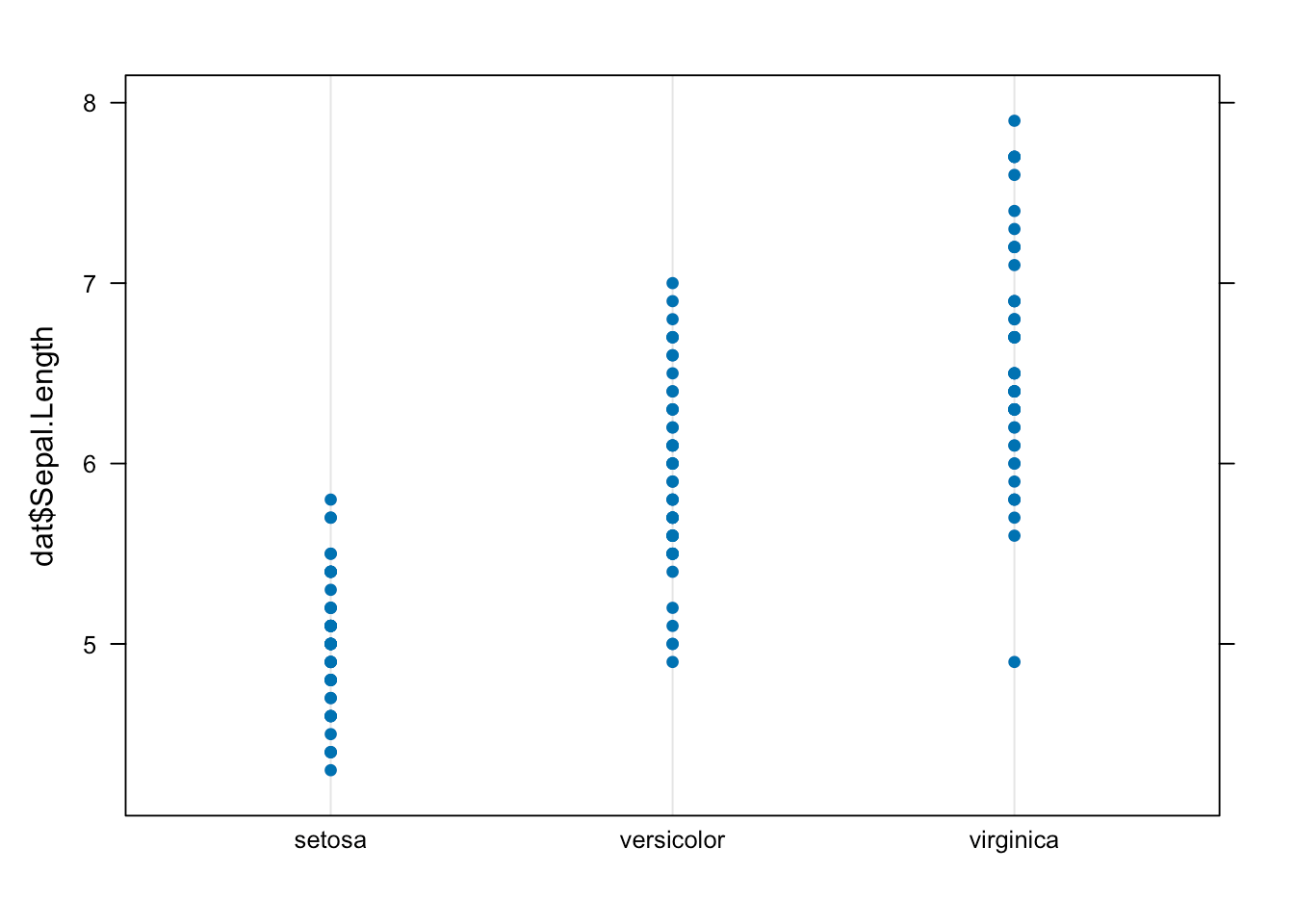Or a QQ-plot with confidence bands with the qqPlot() function from the {car} package:

library(car) # package must be installed first
qqPlot(dat$Sepal.Length)##  132 118 If points are close to the reference line (sometimes referred as Henry’s line) and within the confidence bands, the normality assumption can be considered as met. The bigger the deviation between the points and the reference line and the more they lie outside the confidence bands, the less likely that the normality condition is met. The variable Sepal.Length does not seem to follow a normal distribution because several points lie outside the confidence bands. When facing a non-normal distribution, the first step is usually to apply the logarithm transformation on the data and recheck to see whether the log-transformed data are normally distributed. Applying the logarithm transformation can be done with the log() function. In {ggplot2}: # method 1 qplot(sample = Sepal.Length, data = dat)# method 2 ggplot(dat, aes(sample = Sepal.Length)) + stat_qq()## By groups For some statistical tests, the normality assumption is required in all groups. One solution is to draw a QQ-plot for each group by manually splitting the dataset into different groups and then draw a QQ-plot for each subset of the data (with the methods shown above). Another (easier) solution is to draw a QQ-plot for each group automatically with the argument groups = in the function qqPlot() from the {car} package: qqPlot(dat$Sepal.Length, groups = dat$size)In {ggplot2}: qplot( sample = Sepal.Length, data = dat, col = size, shape = size )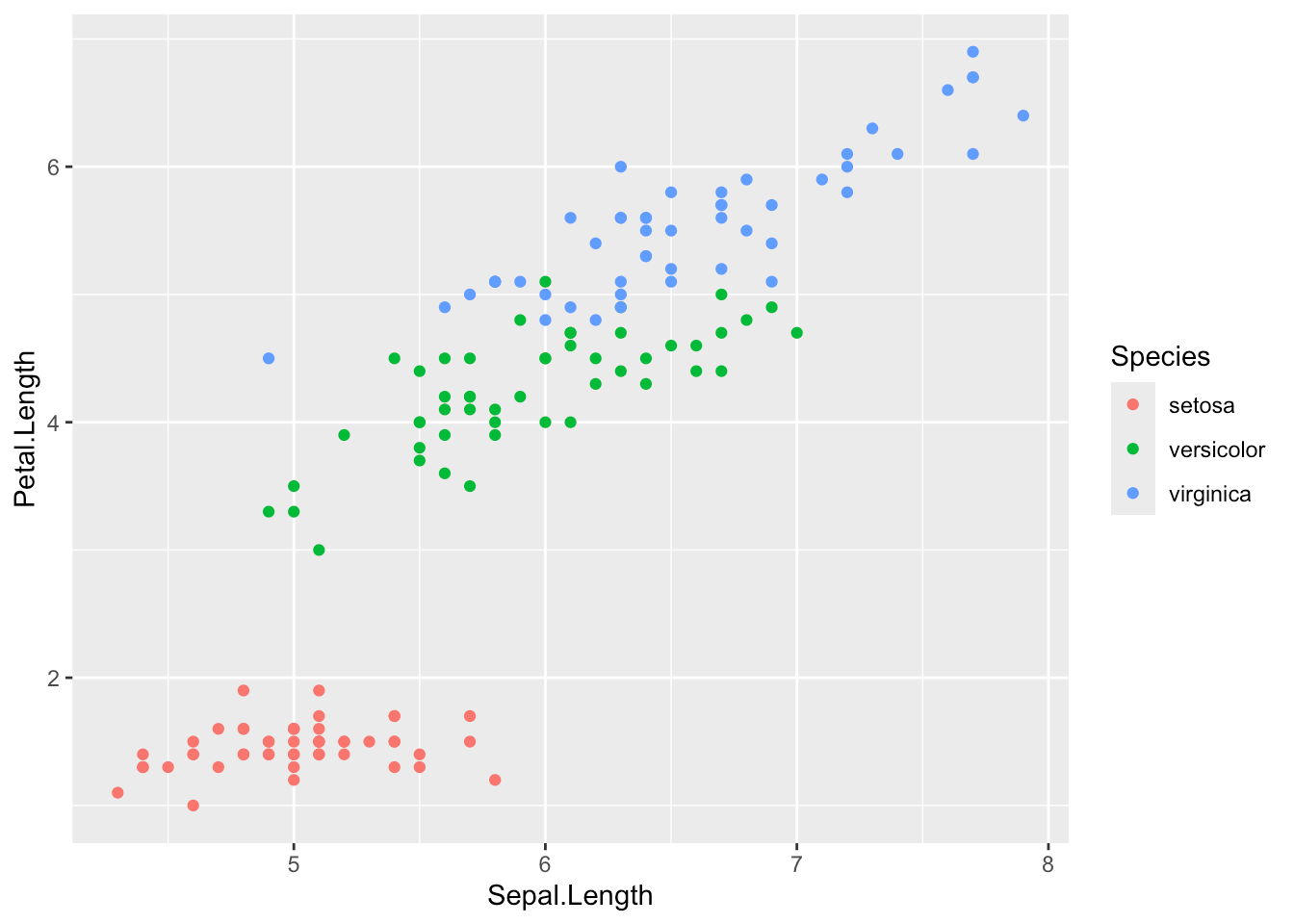It is also possible to differentiate groups by only shape or color. For this, remove one of the argument col or shape in the qplot() function above. # Density plot Density plot is a smoothed version of the histogram and is used in the same concept, that is, to represent the distribution of a numeric variable. The functions plot() and density() are used together to draw a density plot: plot(density(dat$Sepal.Length))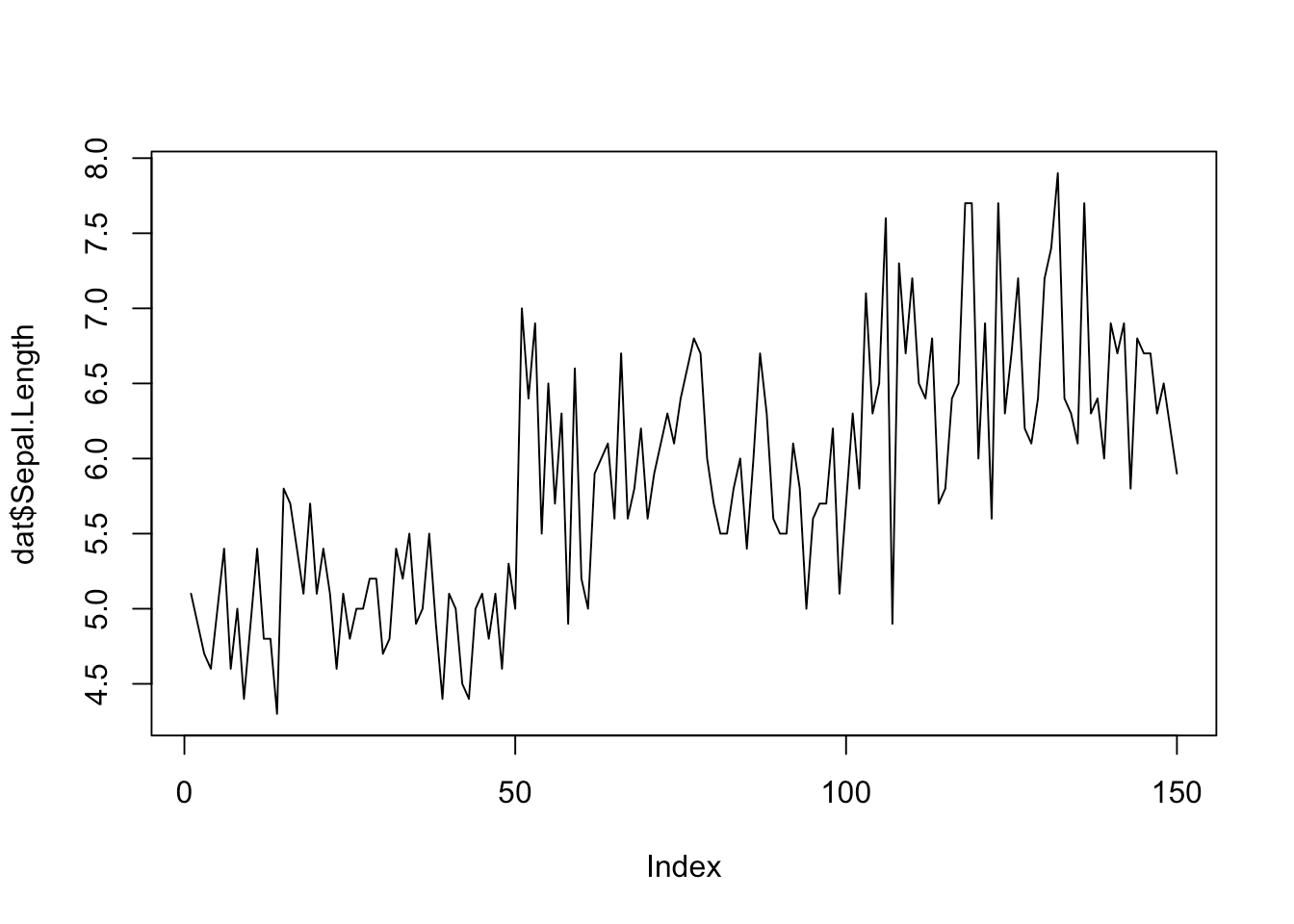In {ggplot2}:

ggplot(dat) +
aes(x = Sepal.Length) +
geom_density()Thanks for reading. I hope this article helped you to do descriptive statistics in R. If you would like to do the same by hand or understand what these statistics represent, read the article “Descriptive statistics by hand”. As always, if you find a mistake/bug or if you have any questions do not hesitate to let me know in the comment section below, raise an issue on GitHub or contact me. Get updates every time a new article is published by subscribing to this blog.

1. Normality tests such as Shapiro-Wilk or Kolmogorov-Smirnov tests can also be used to test whether the data follow a normal distribution or not. However, in practice, normality tests are often considered as too conservative in the sense that sometimes a very limited number of observations may cause the normality condition to be violated. For this reason, it is often the case that the normality condition is verified only based on histograms and QQ-plots.